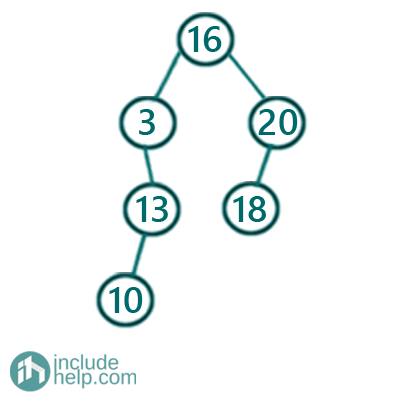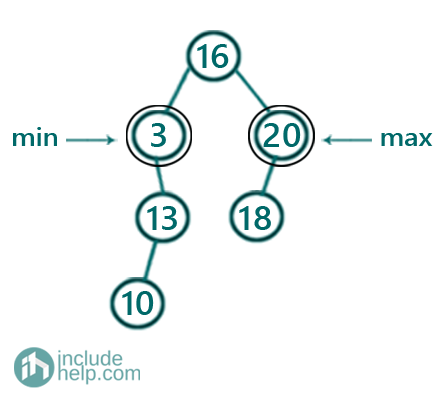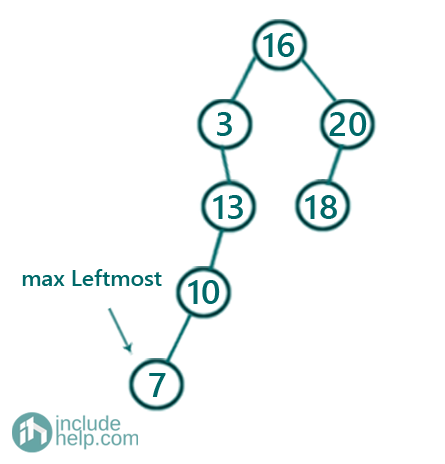# Find the Minimum and Maximum node in a Binary Search Tree

In this article, we are going to see how to find minimum and maximum from a Binary Search Tree?
Submitted by Radib Kar, on October 26, 2020

For example, say the BST is like below:So, the minimum node is 3 and the maximum one is 20,The algorithm is pretty simple. The minimum node will be the leftmost node and the maximum is the rightmost one. But don’t get the word "leftmost" & "rightmost" wrong. The below example may reveal a bit more your confusion if we add one more node with value 7 to the existing tree then the tree will be like:So here the 7 seems to be leftmost one and as per our discussion, it should be the minimum. But it's not as we can see 3 is the minimum still.

So what does "leftmost" or "rightmost" mean here? Leftmost means we will continue to go left starting from left if there is a left child else we will stop and return the current value. Same for the rightmost. We will move to right starting from the root if there is a right child else we will stop and return the current node value.

So, to find the minimum

We will start from root 16

It has a left child so we move to the left

Current node value is 3

There is no left child of current node 3 and hence we stop and return 3 which is our minimum in the binary search tree.

To find the maximum

We will start from root 16

It has a right child so we move to the right

Current node value is 20

There is no right child of node 20 and hence we stop and return 20 which is our maximum in the binary search tree

Below is the C++ implementation:

```#include <bits/stdc++.h>
using namespace std;

// tree node is defined
class TreeNode {
public:
int val;
TreeNode* left;
TreeNode* right;
TreeNode(int data)
{
val = data;
left = NULL;
right = NULL;
}
};

int minValue(TreeNode* root)
{
TreeNode* cur = root;
while (cur->left)
cur = cur->left;

return cur->val;
}

int maxValue(TreeNode* root)
{
TreeNode* cur = root;
while (cur->right)
cur = cur->right;

return cur->val;
}

int main()
{
TreeNode* root = new TreeNode(16);
root->left = new TreeNode(3);
root->left->right = new TreeNode(13);
root->left->right->left = new TreeNode(10);
root->right = new TreeNode(20);
root->right->left = new TreeNode(18);

cout << "Minimum value in the BST is: " << minValue(root) << endl;
cout << "Maximum value in the BST is: " << maxValue(root) << endl;

return 0;
}
```

Output:

```Minimum value in the BST is: 3
Maximum value in the BST is: 20
```

Languages: » C » C++ » C++ STL » Java » Data Structure » C#.Net » Android » Kotlin » SQL
Web Technologies: » PHP » Python » JavaScript » CSS » Ajax » Node.js » Web programming/HTML
Solved programs: » C » C++ » DS » Java » C#
Aptitude que. & ans.: » C » C++ » Java » DBMS
Interview que. & ans.: » C » Embedded C » Java » SEO » HR
CS Subjects: » CS Basics » O.S. » Networks » DBMS » Embedded Systems » Cloud Computing
» Machine learning » CS Organizations » Linux » DOS
More: » Articles » Puzzles » News/Updates